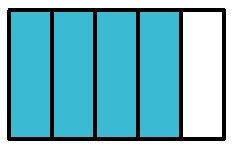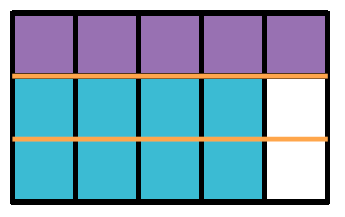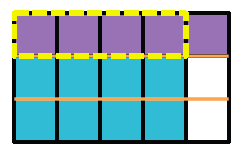### Home > CC2 > Chapter 4 > Lesson 4.3.2 > Problem4-100

4-100.

Multiply $\frac{4}{5}\cdot\frac{1}{3}$. You may want to show your thinking with a diagram.

Shade in $\frac{4}{5}$ of the diagram  A rectangle divided into 5 equal vertical sections with 4 shaded blue.

Shade in $\frac{1}{3}$ of the diagram  The same rectangle divided into 3 equal rows with  the top row (5 squares) shaded purple.

See diagram at right.  The same rectangle with the 4 left top purple sections highlighted.